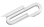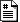Spring 2018

# C

## Feb 1

Snow!

We continued the discussion of pointers and memory and sizeof().

You need to be careful with sizeof() - for memory allocated with malloc , it doesn't know the size of the array.

See the attached stuff.c where we were looking at some of this stuff.

In this case, sizeof(a) does give the total number of bytes that a points at, because when we declared it, the compiler knows at compile time that it's a pointer to 9 integers, which takes 9*4=36 bytes of space.

But sizeof(b) just returns the space needed to store a pointer. The declaration of b is that its an (int*) which is a pointer to an int, and storing a pointer takes 8 bytes (on a 64bit computer).

So you cannot use sizeof to figure out how many numbers you can store as b, b, b, ... .

softmaple:Desktop mahoney$make stuff cc stuff.c -o stuff softmaple:Desktop mahoney$ ./stuff
-- stuff --
a = 0x10d341020
&a = 0x10d341020
a = 1
sizeof(int) = 4
&a = 0x10d341024
sizeof(a) = 36
sizeof(a) = 4
sizeof(b) = 8
sizeof(b) = 4
softmaple:Desktop mahoney\$

/*  stuff.c
*
*/

#include <stdio.h>
#include <stdlib.h>

#define MAX_SIZE 100

int a[] = {1,2,3,4,5,6,7,8,9};

char name = "George Martin";

int c[MAX_SIZE];

int main(){

int* b = malloc(100*sizeof(int));               // space for 100 integers

char* inputbuffer;

inputbuffer = malloc(1000*sizeof(char));  // space for 1000 characters

printf("-- stuff --\n");

printf(" a = %p \n", a);
printf(" &a = %p \n", &a);
printf(" a = %i \n", a);
printf(" sizeof(int) = %lu \n", sizeof(int));
printf(" &a = %p \n", &a);
printf(" sizeof(a) = %lu \n", sizeof(a));
printf(" sizeof(a) = %lu \n", sizeof(a));

b = 10;
b = 20;
printf(" sizeof(b) = %lu \n", sizeof(b));
printf(" sizeof(b) = %lu \n", sizeof(b));

return 0;
}

https://cs.marlboro.college /cours /spring2018 /jims_tutorials /web_tech /c_feb1
## attachmentslast modified sizestuff.c Tue Dec 05 2023 08:49 am 790B